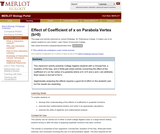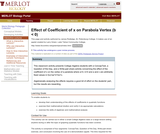Updating search results...

# 5 Results

View
Selected filters:
• Starting Point (SERC)Conditional Remix & Share Permitted
CC BY-NC-SA
Rating
0.0 stars

This classroom activity presents College Algebra students with a ConcepTest, a Question of the Day, and a Write-pair-share activity concerning the effect of the coefficient of x^0 (i.e., the constant, c) on the vertex of a parabola where a and b are arbitrarily fixed values in f(x)=ax^2+bx+c.

Subject:
Mathematics
Material Type:
Activity/Lab
Assessment
Provider:
Science Education Resource Center (SERC) at Carleton College
Provider Set:
Starting Point (SERC)
Author:
James J. Rutledge
08/28/2012Conditional Remix & Share Permitted
CC BY-NC-SA
Rating
0.0 stars

This classroom activity presents College Algebra students with a ConcepTest, a Question of the Day, and a Write-pair-share activity concerning the effect of the coefficient of x^2 on the vertex of a parabola where a>0, b>0 and b and c are fixed values in f(x)=ax^2+bx+c.

Subject:
Mathematics
Material Type:
Activity/Lab
Assessment
Provider:
Science Education Resource Center (SERC) at Carleton College
Provider Set:
Starting Point (SERC)
Author:
James J. Rutledge
08/28/2012Conditional Remix & Share Permitted
CC BY-NC-SA
Rating
0.0 stars

This classroom activity presents College Algebra students with a ConcepTest, a Question of the Day, and a Write-pair-share activity concerning the effect of the coefficient of x^2 on the vertex of a parabola where a<0, b>0 and b and c are fixed values in f(x)=ax^2+bx+c.

Subject:
Mathematics
Material Type:
Activity/Lab
Assessment
Provider:
Science Education Resource Center (SERC) at Carleton College
Provider Set:
Starting Point (SERC)
Author:
James J. Rutledge
08/28/2012Conditional Remix & Share Permitted
CC BY-NC-SA
Rating
0.0 stars

This classroom activity presents College Algebra students with a ConcepTest, a Question of the Day, and a Write-pair-share activity concerning the effect of the coefficient of x on the vertex of a parabola where a>0, b>0 and a and c are fixed values in f(x)=ax^2+bx+c.

Subject:
Mathematics
Material Type:
Activity/Lab
Assessment
Provider:
Science Education Resource Center (SERC) at Carleton College
Provider Set:
Starting Point (SERC)
Author:
James J. Rutledge
08/28/2012Conditional Remix & Share Permitted
CC BY-NC-SA
Rating
0.0 stars

This classroom activity presents College Algebra students with a ConcepTest, a Question of the Day, and a Write-pair-share activity concerning the effect of the coefficient of x on the vertex of a parabola where a>0, b<0 and a and c are fixed values in f(x)=ax^2+bx+c.

Subject:
Mathematics
Material Type:
Activity/Lab
Assessment
Provider:
Science Education Resource Center (SERC) at Carleton College
Provider Set:
Starting Point (SERC)
Author:
James J. Rutledge